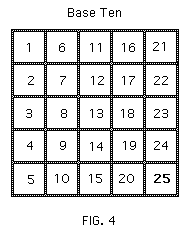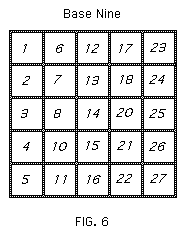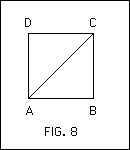# Heinrich Tietze on Numbers, Part 2

Heinrich Tietze lectured on mathematics to students at the University of Munich who were studying a wide variety of different subjects. These students came from every faculty of the University. Tietze's aim was to talk about those mathematical topics which would convey the beauty of the subject, but at the same time required little background (as most of the students had very mathematical knowledge).

Below is a version of the second part of a lecture he gave on Numbers and Counting. This lecture formed the basis for a chapter in his book Gelöste und ungelöste mathematische Probleme aus alter und neuer Zeit which appeared in English translation as Famous Problems of Mathematics: Solved and Unsolved Mathematical Problems from Antiquity to Modern Times (1965).

For the first part of Tietze's lecture, follow this link: Tietze on Numbers 1

### NUMBERS AND COUNTING Part 2

Another essential property of a number, independent of its designation, is that of being a square, that is the product of a number by itself: e.g. twenty-five = five times five = five squared. The reason is that the statement "N is a square" merely means that there is a square which can be partitioned into N subsquares in the way shown in Figs. 4, 5, and 6for N twenty-five. Whether the subsquares are numbered relative to the decimal system (Fig. 4), or the duodecimal system (Fig. 5), or the nonary system (Fig. 6) has no bearing on the fact that the number of subsquares is a square number.

The property of being either a composite number or a prime is also an essential property. On the other hand, a "round" number, a number which ends in one or more zeros, is clearly dependent on the choice of base. The celebration of a 70th or 80th birthday, or a 50th wedding anniversary, the designation of centuries as historical units of comparison, are also tied to a system based on the number ten. In the duodecimal system, entirely different numbers, those divisible by 12, would be "round."

We have dwelt on the role of the number ten in our numerical system to show that ten has no essential mathematical properties which make it basic to counting, but that on the contrary any other positive whole number (greater than one) would do just as well. What is decisive, is the positional principle on which all our representational systems rest, no matter what the base. This positional principle represents an arbitrary number by digits, each of which is assigned a positional value; a special symbol, our zero, is required for the positions not occupied by digits.

The reader has probably already asked himself where the mathematical problem is in all this? In ancient times or among primitive peoples, the development of a systematic method of counting and of representing numbers was in fact a problem of great cultural importance, whether or not it was consciously posed. Methods of counting were evolved gradually, by no one man. They culminated in the positional principle and in the choice of a base, which made it possible to represent any number, no matter how large, with a limited number of symbols (digits). This systematic representation of numbers is intimately linked with the perception - already developed by Archimedes in his grains of sand theory - that the sequence of numbers 1, 2, 3, - - - is infinite. The difficulty in evolving the positional system (which children now take for granted) is best illustrated by the history of zero, i.e. the introduction of a symbol for an unoccupied position - a symbol which, as we know, the Romans lacked. To grasp the progress represented by the decimal system and its positional system (which gradually spread over Europe only after the year 1000), consider the problem of conducting all our monetary, insurance, statistical and other numerical affairs in Roman numerals. Compare the time required to do a simple problem in addition in the decimal system with that required by the same problem using Roman numerals:
 146      CXLVI
1993    MCMXCII
500          D
204       CCIV
59        LIX
140        CXL
98     XCVIII
301       CCCI
----   --------
3441   MMMCDXLI

How much more time, energy and personnel would be necessary in ordinary business computation without the positional system.

It would not, then, make a great deal of difference to counting and calculating if man had been born with six fingers on each hand. It is not the preference accorded the number ten which matters, but rather the creation of a positional system for representing numbers, which is of decisive significance for our culture.

The origin of whole numbers in the counting process was the first mathematical activity of man. In addition, as long ago as the Babylonians and the Egyptians, man already knew fractions. A developed arithmetic dating back to 2000 B.C., with addition, subtraction, multiplication and division of fractions, was found in the Rhind papyrus. Later, the domain of numbers was extended in three ways, but each extension had to overcome many obstacles. The three generalizations which successively broadened the concept of number were those of negative, irrational, and finally of imaginary numbers.

If one follows the vicissitudes suffered by these numbers before they gained full maturity and recognition, one finds in each case the same childhood diseases and the same adolescent difficulties in the transition to a ripe, fully developed theory, First, there were tentative trials of the new numbers, whose foundations were uncertain; then a growing familiarity with them on the one hand, and a distinct aversion towards them on the other - if indeed their existence was recognized at all; at the same time a defence of the new acquisition, often on faulty grounds, was carried on by its champions.

Those who remember their own introduction to negative, then irrational and perhaps imaginary numbers, will agree that the stages in their learning process were analogous to those of the historical development of the subject and that the process is comparable to the well-known biological principle: ontogeny recapitulates phylogeny. We will not discuss classroom reminiscences of imaginary numbers, even though these numbers are very important in the development of other branches of mathematics. We must certainly recall our own resistance to the rule for multiplying negative numbers: -1 × -1 = +1 (minus times minus equals plus). As for the irrational numbers, the high schools are forced to forego teaching them on a really rigorous basis, because this is much too difficult. High schools have to try to get by as cheaply as possible without making the gifted students feel that they are being stuffed with material merely because it is prescribed, and hence to become disenchanted with the subject because of its apparent lack of rigour.

A major difficulty in understanding the new kinds of numbers - as evidenced both by their historical development and the learning process of the student - is the fact that their main function is no longer that of counting, but of measuring. Both fractions and negative numbers, however, do not lose their counting function completely. If I take several apples, about equal in size, and cut each of them into three parts, and then take five of these parts, the fraction 5 /3 still involves counting in the sense that 5 is a numerator or numberer. However, the part has replaced the whole as a unit. Negative numbers are used with positive ones in counting outgo and intake, so that commercial arithmetic has played a substantial role in the extension of the number domain. But the fraction 5 /3 can also be interpreted as the measurement of a length (by dividing the length into three equal parts and then considering five such parts side by side). Negative and positive numbers can also be used to measure lengths by taking a fixed point on a line and specifying that measurements to the right of the point are positive and to the left negative. An example is the temperature scale of a thermometer.

Before discussing the measuring functions of fractions and negative numbers any further, we must consider irrational numbers. A rigorous foundation for these numbers was not developed until the latter part of the 19th century, although their origin goes back to classical antiquity. Although we cannot fully treat the subject of irrational numbers here, we can indicate the nature of the problem solved by the introduction of irrational numbers.Consider Fig. 7, in which $AB$ is the unit of length and the length of $AC$ is 5 /3 . Consequently, 1 /5 of $AC$ is equal to 1 /3 of $AB$, that is $AC : AB$ as 5 : 3. Hence, the two segments are in the ratio of two whole numbers. In other words, $AC$ and $AB$ have a common measure, $AE$ (of which they are both multiples). Therefore, $AC$ and $AB$ are said to be commensurable.

The Greeks perceived that there are segments which are not in the ratio of two whole numbers, and therefore have no common measure or are incommensurable. The most familiar example is the one given by Euclid in the 10th book of his Elements (Fig. 8).The diagonal $AC$ and the side $AB$ of the square $ABCD$ are incommensurable, since the contrary assumption leads to a contradiction. For if $AC$ and $AB$ have a common measure, it is a segment which can be marked off exactly $m$ times on $AC$ and $n$ times on $AB$, with $m$ and $n$ suitable positive whole numbers; then the $m$th part of $AC$ is equal to the $n$th part of $AB$ and $AC : AB$ as $m : n$. It can be shown that this implies that
$m^{2} = 2n^{2}$.

Further, it can be proved that whole numbers $m$ and $n$ cannot satisfy this equation. Hence there cannot be two whole numbers $m$ and $n$ such that $AC$ and $AB$ are in the ratio $m : n$.

[We can cheek this by carefully measuring the side and diagonal of a square. If $AB$ is divided into 1000 parts, measurement of $AC$ will yield 1414 of these parts so that $AC : AB$ is as 1414 : 1000, or 707 : 500. But, if $m$ = 707, and $n$ = 500,
$m^{2} = 499849$

and
$2n^{2} = 2 \times 250000 = 500000$,

so that $m^{2}$ and $2n^{2}$ are only approximately, but not exactly equal, as they must be if $m : n$ is to be the exact ratio of the diagonal to the side of a square.

One must thoroughly understand that the spirit of classical Greek mathematics was based on exactitude and went far beyond the practical considerations of surveying. Therefore a sharp distinction must be made between approximate, and absolutely exact relationships. Only a rigorously exact mathematics could make the distinction between commensurable and incommensurable segments - a distinction which would make no sense in practical measurements.]

Therefore, if $AB$ is the unit of length, the length of the segment $AC$ cannot be represented by any fraction $\large\frac{m}{n}$. Nevertheless, the need to assign numbers to the ratios of any two segments (commensurable or not) grew more and more imperative. This could be done only by extending the domain of fractions (rational numbers) by introducing new numbers-those we. call irrational.

Greek mathematics could compare two segments as equal (or larger or smaller); what it lacked was the concept of assigning numbers to lengths of segments incommensurable with a prescribed unit segment. While now we can simply say: If the side $AB$ is the unit of length, the length of the diagonal $AC$ is equal to √2, this would have been impossible for the Greeks because √2 does not exist in the domain of rational numbers.Another example is the problem of adding $AE = AC$ to AB (Fig. 9). We can write the sum $EB$ as 1 + √2; the Greeks could add the two segments geometrically, but could not add them arithmetically.

The extension of the number system was required not only for geometric measurements, but also for the solution of algebraic equations. As a result, beginning with the Arab mathematicians, the use of irrational numbers spread after 1000 A.D. It was Michael Stifel (1487-1567) who gave them the name of irrational numbers. But it was not until the 19th century that rigorous theories of irrational numbers were developed. During the second half of the century, a period of critical activity and great discoveries, many leading mathematicians became interested in building a solid foundation for mathematics. Various theories of irrational numbers were evolved; among them the theory of Dedekind, which since then has been preferred by an ever growing number of mathematicians. Dedekind's theory of irrational numbers is too technical for discussion here, but we do want to say a few words about this great thinker.

Whoever is willing to agree that the outlook of all branches of mathematics is essentially determined by the ideas of outstanding investigators and the new concepts introduced by them, must recognize in Dedekind one of those creative spirits who have given modern mathematics its characteristic stamp. Dedekind's work (the theory of ideals) was in a field in which Gauss had been a pioneer; it was algebraic in origin and dealt among other things with problems of factorization analogous to the familiar problem of factoring a number into its prime factors, e.g.: 84 = 2.2.3.7. Kummer (1810-1893) had already illuminated part of this field. Dedekind's theory went on to embrace the whole field and was so profound that it determined the development not only of algebra but of other branches of mathematics, and will no doubt continue to do so for a long time.

Dedekind's achievements had to do not with calculating but with the analysis of concepts, which made it difficult at the beginning for his contemporaries to enter into his new conceptual world. This was true even in the case of the theory of irrational numbers. Added to this barrier was the fact that the need for such a rigorous, systematic treatment of irrational numbers, first recognized by Dedekind, was by no means generally realized. He explained this need in 1858, in a lecture given while he was a Professor at Zurich. By late autumn of that same year he had evolved a systematic theory, but it was not until 1872 that he published it, under the stimulus of the appearance of other theories of irrational numbers. Even then the new concepts were slow to influence the foundations of arithmetic and were still rejected by many.

Significant changes in mathematics and the great impetus they gave to new surges of development were conceived by a man whose outer life was so uneventful that it can be recounted in a few lines.

Richard Dedekind was born in Brunswick (the birthplace of the great Gauss), 6 October 1831, where he lived until he was 19. After twelve years of study and teaching elsewhere, he returned to stay until his death in his 85th year. In 1850 he went to continue his studies, which he had begun at the Collegium Carolinum in 1848, in Göttingen. He received his doctorate from Göttingen in 1852 and in 1854 became a lecturer. In 1858 he was made Professor at the Federal Institute of Technology at Zurich, and in 1862 was called to the Technische Hochschule in Brunswick.